Matlab and Bryce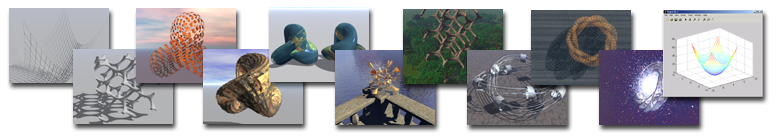I enjoy visualizing mathematical surfaces and simulation results in Matlab, but sometimes I want more than just a mesh - a bit of texturing, nice surroundings, a sky and maybe some photorealistic decorations. That was why I wrote a few simple scripts to export meshes and isosurfaces from Matlab to the Alias/Wavefront OBJ format, which can be read by Bryce.

Why Obj? Because it is simple, and can be read by many programs and converters.

Why Matlab? Because it is a great environment for doing math, simulation and numerical hacking even if the language itself is crude, from a computer science standpoint.

Saving a mesh

Saveobjmesh.m saves a rectangular mesh of points as an Obj file.

Example:

[u,v]=meshgrid(-6:0.5:6,-6:0.5:6);

x=u; y=v; z=u.^2+v.^2;

mesh(x,y,z)

saveobjmesh('parab.obj',x,y,z)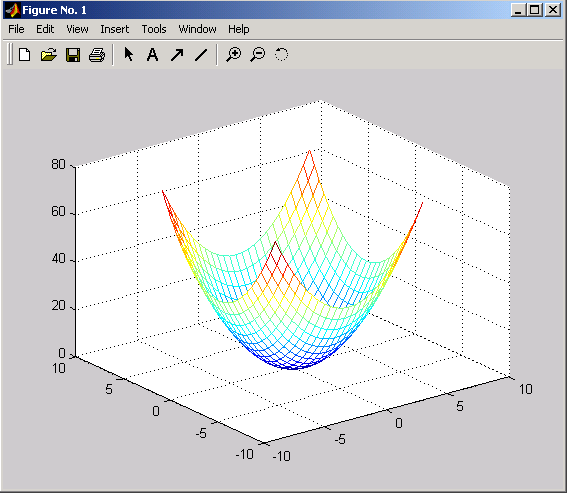This can then be imported into Bryce (File->Import Object). In this case a bit of scaling might be needed.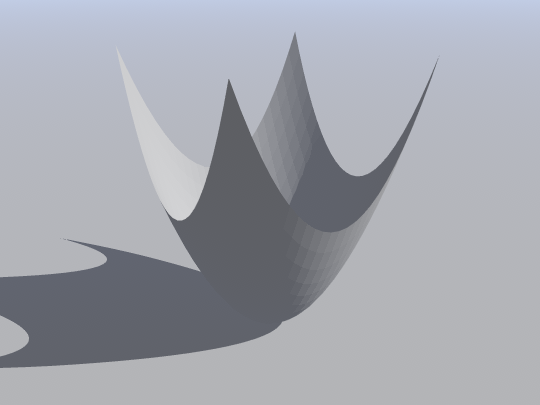The default version does not include any normal information, making the polygon edges look hard. Saveobjmesh allows you to add normals, although the smoothing in Bryce makes a nice job of calculating them anyway.

Example:

[u,v]=meshgrid(-6:0.5:6,-6:0.5:6);

x=u; y=v; z=u.^2+v.^2;

mesh(x,y,z)

nx=-2*x; ny=-2*y; nz=1+nx*0;

saveobjmesh('parab.obj',x,y,z,nx,ny,nz)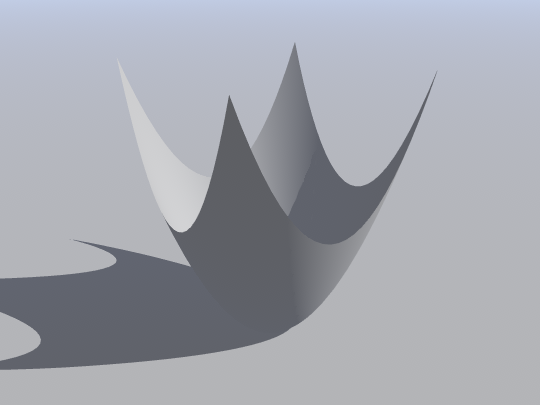Sometimes one wants a literal mesh rather than a polygonal surface. I wrote a version of saveobjmesh, saveobjmeshgrid.m, that instead of each quadrilateral draws four smaller quadrilaterals around a hole, producing a nice mesh-like surface.

saveobjmeshgrid('parab.obj',x,y,z,0.1)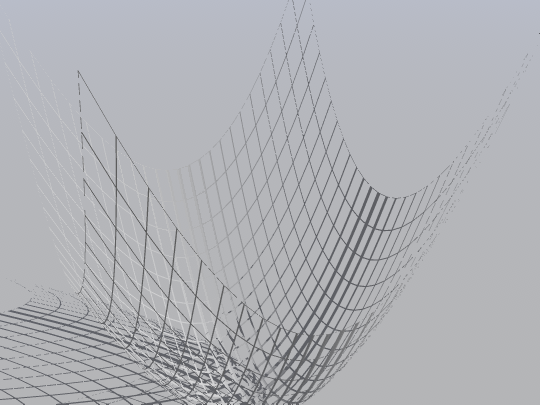Saving Isosurfaces

Isosurfaces are extremely versatile (as Povray 3.5 demonstrates). It is surprisingly easy to save them from Matlab, since the isosurface command already does much of the job. The vertface2obj.m function saves the vertex and face lists the isosurface command generates.

Example:

a=2*pi;

s=2*pi/60;

[x,y,z]=meshgrid(-a:s:a,-a:s:a,-a:s:a);

cx = cos(2*x);

cy = cos(2*y);

cz = cos(2*z);

u = 10.0*(cos(x).*sin(y) + cos(y).*sin(z) + cos(z).*sin(x))- 0.5*(cx.*cy + cy.*cz + cz.*cx) - 14.0;

[f,v]=isosurface(u,0);

vertface2obj(v,f,'gyroid.obj')

The first lines creates a function with a nice gyroid lattice structure (formula from The Scientific Graphics Project). Then the u=0 isosurface is calculated, which produces a list of vertices and faces. These are saved with vertface2obj. In this case the mesh resolution is rather high in order not to miss any pieces.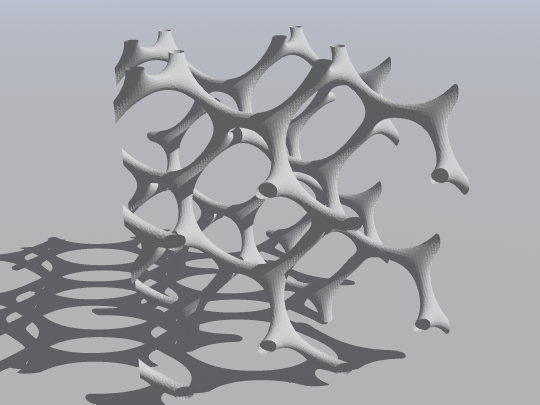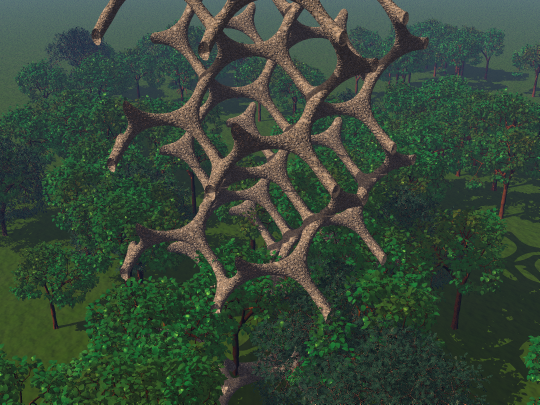Another example is the Barth sextic

s=0.05;

[x,y,z]=meshgrid(-2:s:2,-2:s:2,-2:s:2);

t = 0.5*(1 + sqrt(5));

u= 4*(t^2* x.^2 - y.^2).*(t^2.* y.^2 - z.^2).*(t^2 .* z.^2 - x.^2) - (1+2*t)*(x.^2 + y.^2 + z.^2 - 1).^2;

[f,v]=isosurface(u,0);

vertface2obj(v,f,'barth.obj')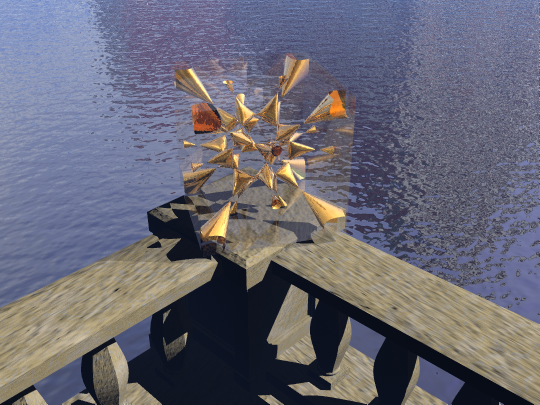Here it is placed on a verandah overlooking a canal.

Some Further Examples

Once the object has been imported, one can do all the usual fun Bryce stuff with it.

Horn Gates=2*pi/100;

[u,v]=meshgrid(0:s:(2*pi),0:s:(2*pi));

k=0.5;

t=.05*(2*pi-u).*(1+sin(v*2+u*3)./(1+sin(u*4).^2));

x=cos(u*k).*(1+t.*cos(v));

y=sin(u*k).*(1+t.*cos(v));

z=  t.*sin(v);

In this case I made one "horn" mesh and a smaller straight mesh and imported them into Bryce. Then I multireplicated the smaller straight mesh in a circle around the foot of the big horn to make the base. Then I grouped them and made a mirrored copy.

Knots

Plotting knots becomes relatively easy, at least toroidal knots:

su=2*pi/150;

sv=2*pi/20;

[u,v]=meshgrid(0:su:(2*pi),0:sv:(2*pi));

p1=2; % Turns around origin

p2=3; % Twists around itself

x=r1*cos(u*p1) + r2*cos(u*p1).*cos(u*p2) +r3*cos(u*p1).*sin(v);

y=r1*sin(u*p1) + r2*sin(u*p1).*cos(u*p2) +r3*sin(u*p1).*sin(v);

z=r2*sin(u*p2)+r3*cos(v);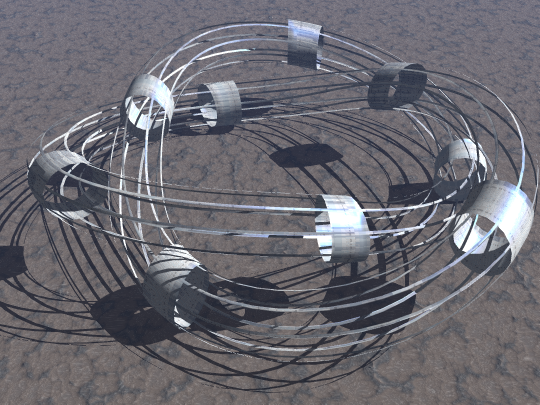A threefoil knot with the Bryce default metal grid texture, mapped parametrically. Since the u,v coordinates of the above script are not locally orthogonal, a nice twistiness is apparent in the texture.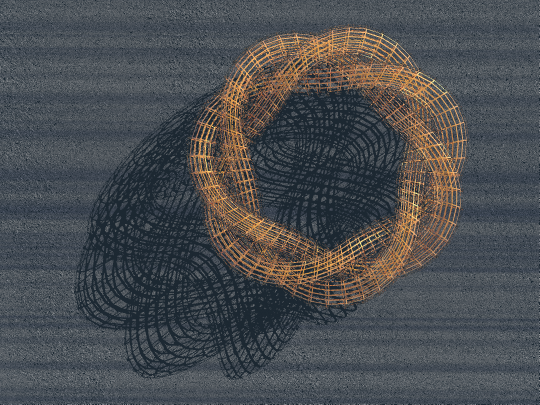This time the mesh is a real grid (made by saveobjmeshgrid) of a (3,7) knot.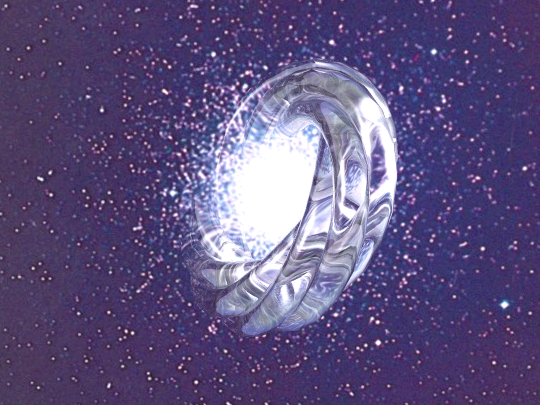A knot where the tube radius produces self-intersections. The globular cluster is imagemapped on a square, and then the glass-textured knot placed in front. No postprocessing was needed.

Exploded meshes

A cool effect is to shrink each individual polygon, creating an "exploded" appearance. The script explodemesh.m takes a mesh and shrinks each quadrilateral towards it's center of mass by a constant factor.

Here it is applied to Boy's surface:

ss=pi/100;

[s,t]=meshgrid(0:ss:pi,0:ss:pi);

x=cos(t).*sin(s);

y=sin(t).*sin(s);

z=cos(s);

f=1/2*((2*x.^2-y.^2-z.^2) + 2*y.*z.*(y.^2-z.^2) + z.*x.*(x.^2-z.^2)+x.*y.*(y.^2-x.^2));

g=sqrt(3)/2*((y.^2-z.^2) + z.*x.*(z.^2-x.^2) + x.*y.*(y.^2-x.^2));

h=((x+y+z).*((x+y+z).^3 + 4*(y-x).*(z-y).*(x-z)))/8;

explodemesh('b.obj',h/8,f,g,0.5);

This way the somewhat tricky interconnections of the surface becomes visible: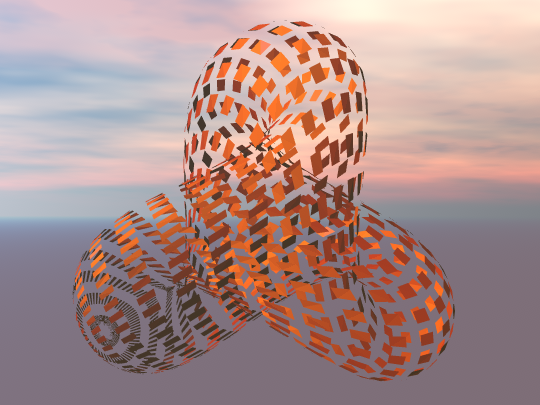By giving a factor larger than one to explodemesh the polygons instead becomes scales: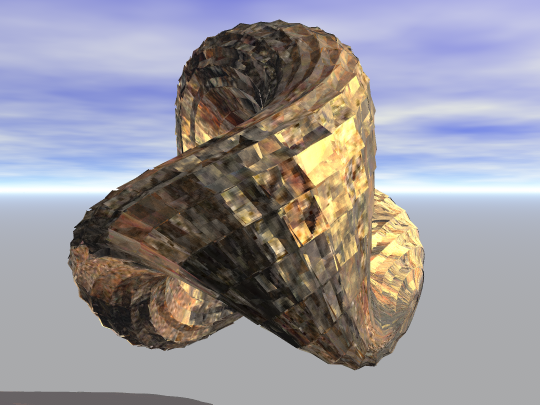As an aside, the parametrization allows one to map the Earth onto the surface. Obviously there has to be something odd going on since it is non-orientable and the Earth's surface certainly is, but it is not easy to see where it is.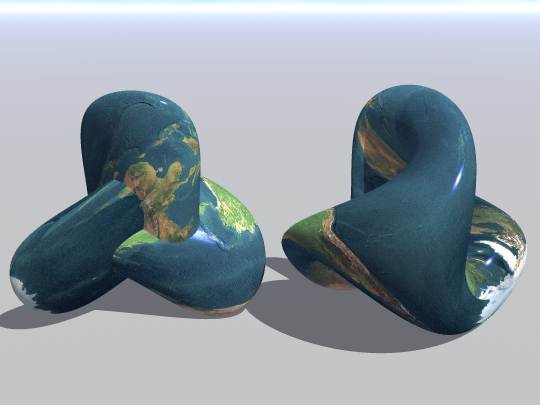The Obj Format

While Obj can handle complex objects involving curves, bezier patches and much more, these scripts merely use the polygonal interface. It goes a long way.

An Obj file is an ascii file with information organized into lines. The important kinds of lines are:

#
comments. Lines starting with # are ignored
v x y z
Vertex. A line starting with a "v" followed by three numbers specify a vertex. Each vertex gets a running number from one and upwards.
vt u v
Texture coordinates. Lines starting "vt" contain the u,v coordinates of a point. Which texture location goes with which geometric location is determined by the face definitions (see below).
vn x y z
Normal coordinates. Defines a normal vector for some point.
f v1[/vt1][/vn1] v2[/vt2][/vn2] ...
Face definition. Followed by a list of numbers specifying vertices ("f 4 5 6" makes a face of vertex 4,5 and 6), a list of number pairs for vertices with texture coordinates ("f 4/1 5/2 6/3" links vertex 4 to texture vertex 1m vertex 5 to 2 and so on) or a list of location, texture and normals ("f 4/1/1 5/2/2 6/3/3").
g name
Group all subsequent faces into a group.
A simple example would be

v 0 0 0

v 1 0 0

v 0 1 0

v 1 1 0

g square

f 1 2 3

f 2 4 3

Which defines a square shape consisting of two triangular faces. The normal is assumed to point upwards if the vertices are passed counterclockwise.

See the links below for further descriptions and specifications.